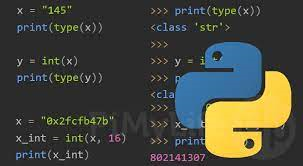# How to Convert All Strings in List to Integer

Often you may need to convert a list of string representation of integers into a list of integers in Python. There are several ways to do this. We will learn how to convert all strings in list to integer in Python.

## How to Convert All Strings in List to Integer

Let us say you have the following list of strings.

`a = ['1', '2', '3']`

Let us say you want to convert it into a list of integers.

`[1, 2, 3]`

Here are the different ways to convert this list of strings into list of integers.

## 1. Using Map

You can use map() function to easily convert each string item into integer in python as shown below.

```list(map(int, a))
[1, 2, 3]```

In Python 2, you can directly use map() function since it already returns a list.

```map(int, a)
[1, 2, 3]```

In both the above commands, map() function maps the int() function on each item of list to convert it into integer.

## 2. Using List Comprehension

You can also use list comprehension to convert list of strings to list of integers as shown below.

```[int(x) for x in a]
[1, 2, 3]```

In the above code, the list comprehension iterates through each item and calls int() function to cast it into an integer and return it.

In this article, we have learnt how to convert all strings in list to integer.